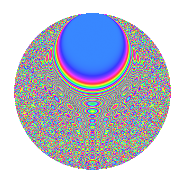# Properties

 Label 3150.2.crLevel 3150 Weight 2 Character orbit cr Rep. character $$\chi_{3150}(361,\cdot)$$ Character field $$\Q(\zeta_{15})$$ Dimension 800 Sturm bound 1440

# Related objects

## Defining parameters

 Level: $$N$$ = $$3150 = 2 \cdot 3^{2} \cdot 5^{2} \cdot 7$$ Weight: $$k$$ = $$2$$ Character orbit: $$[\chi]$$ = 3150.cr (of order $$15$$ and degree $$8$$) Character conductor: $$\operatorname{cond}(\chi)$$ = $$175$$ Character field: $$\Q(\zeta_{15})$$ Sturm bound: $$1440$$

## Dimensions

The following table gives the dimensions of various subspaces of $$M_{2}(3150, [\chi])$$.

Total New Old
Modular forms 5888 800 5088
Cusp forms 5632 800 4832
Eisenstein series 256 0 256

## Trace form

 $$800q + 100q^{4} - 2q^{5} + O(q^{10})$$ $$800q + 100q^{4} - 2q^{5} + 2q^{10} + 6q^{11} + 100q^{16} + 4q^{19} + 4q^{20} - 32q^{22} - 14q^{23} + 48q^{26} - 2q^{28} + 24q^{29} - 6q^{31} - 16q^{34} - 24q^{35} - 20q^{37} - 8q^{38} + 2q^{40} - 4q^{41} - 72q^{43} - 4q^{44} + 12q^{46} + 20q^{47} - 16q^{50} - 16q^{53} + 24q^{55} + 8q^{58} + 8q^{61} - 200q^{64} + 18q^{65} + 32q^{67} - 40q^{68} + 46q^{70} + 4q^{71} - 36q^{73} + 16q^{74} + 32q^{76} - 8q^{77} - 2q^{80} - 64q^{82} + 40q^{83} + 12q^{85} - 12q^{86} - 14q^{88} - 54q^{89} - 4q^{91} - 32q^{92} + 32q^{94} - 32q^{95} + 132q^{97} + 32q^{98} + O(q^{100})$$

## Decomposition of $$S_{2}^{\mathrm{new}}(3150, [\chi])$$ into newform subspaces

The newforms in this space have not yet been added to the LMFDB.

## Decomposition of $$S_{2}^{\mathrm{old}}(3150, [\chi])$$ into lower level spaces

$$S_{2}^{\mathrm{old}}(3150, [\chi]) \cong$$ $$S_{2}^{\mathrm{new}}(175, [\chi])$$$$^{\oplus 6}$$$$\oplus$$$$S_{2}^{\mathrm{new}}(350, [\chi])$$$$^{\oplus 3}$$$$\oplus$$$$S_{2}^{\mathrm{new}}(525, [\chi])$$$$^{\oplus 4}$$$$\oplus$$$$S_{2}^{\mathrm{new}}(1050, [\chi])$$$$^{\oplus 2}$$$$\oplus$$$$S_{2}^{\mathrm{new}}(1575, [\chi])$$$$^{\oplus 2}$$

## Hecke Characteristic Polynomials

There are no characteristic polynomials of Hecke operators in the database